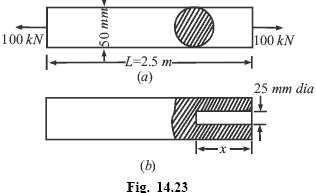# A steel tie rod. 50 mm in diameter and 2.5 m long is subjected to a pull of 100 kN. To what length the rod should be bored centrally so that the total extension will increase by 15 per cent under the same pull, the bore being 25 mm diameter? Take E = 200 GN / m2

Question-AnswerCategory: Strength of MaterialsA steel tie rod. 50 mm in diameter and 2.5 m long is subjected to a pull of 100 kN. To what length the rod should be bored centrally so that the total extension will increase by 15 per cent under the same pull, the bore being 25 mm diameter? Take E = 200 GN / m2Example 1.7. A steel tie rod. 50 mm in diameter and 2.5 m long is subjected to a pull of 100 kN. To what length the rod should be bored centrally so that the total extension will increase by 15 per cent under the same pull, the bore being 25 mm diameter? Take E = 200 GN / m2

Diameter of the steel tie rod = 50 mm = 0.05 m
Length of the steel rod, L = 2.5 m
Magnitude of the pull, P = 100 kN
Diameter of the bore = 25 mm = 0.025 m
Modulus of elasticity, E = 200 × 109 N/m2Let length of the bore be ‘x’
Stress in the solid rod σ = P/A = {(100 × 1000)/[(Π/4)(0.05)2]} = 50.92 × 106 N/m2
Elongation of the rod δL = σL/E = (50.92 × 106 × 2.5) / (200 × 109) = 0.000636m = 0.636mm
Elongation after the rod is bored = 1.15 × 0.636 = 0.731mm
Area of the reduction section = (Π/4) (0.052 – 0.0252) = 0.001472m2
Stress in the reduced section σb = (100 × 1000)/0.001472m2 = 67.93 × 106 N/m2
Elongation of the rod = σ(2.5 – x)/E + σb.x/E = 0.731 × 10–3
= [50.92 × 106 (2.5 – x)]/(200 × 109) + (67.93 × 106.×)/(200 × 109 ) = 0.731 × 10–3 x = 1.12m
Hence length of bore=1.12m